# Find the equation of an ellipse

• Matejxx1
I did give a=b=8 as one solution, making it a circle, with c=0.I would be a little surprised if this is the case.

## Homework Statement

Hello, my friend asked my If I could help him with this problem. However I just can't seem to find a way to solve this.
Ellipse
Focus(2,2)
vertex(2,-6)
Point(26/5,2)
a+e=8
find the equation of the ellipse

## Homework Equations

(x-m)^2/a^2+(y-n)^2/b^2=1
Center(m,n)
a=moyor axis
b=minor axis

## The Attempt at a Solution

I will post pictures of my work as I don't yet know how to use math syntax on the internet.
I would really appreciate if you could find my mistake. I tried duing everything, however I can't seem to get the point(26/5,2) to be on my ellipse. Everything else looks fine
The order of which I did the problem is represented by roman numbers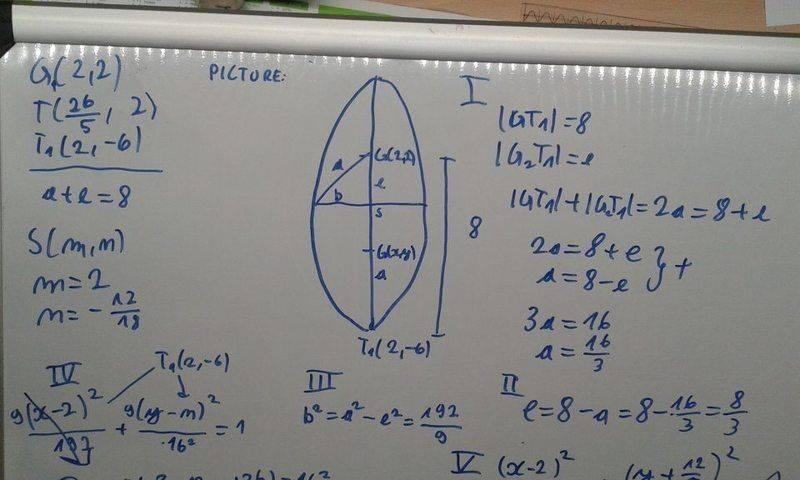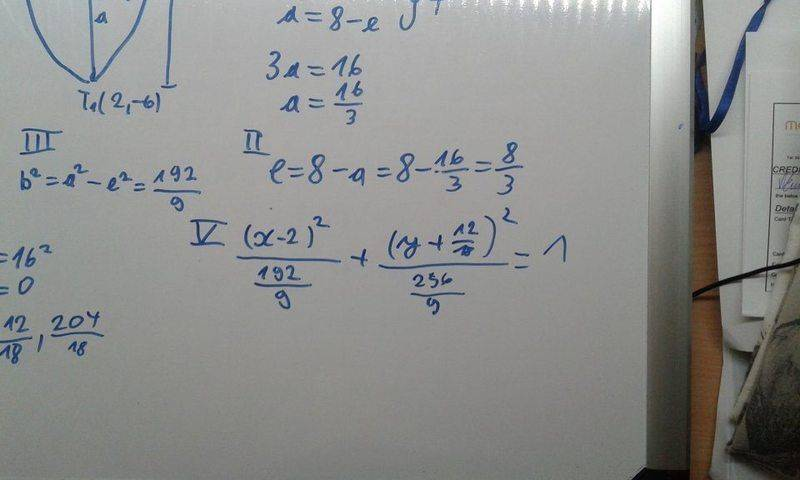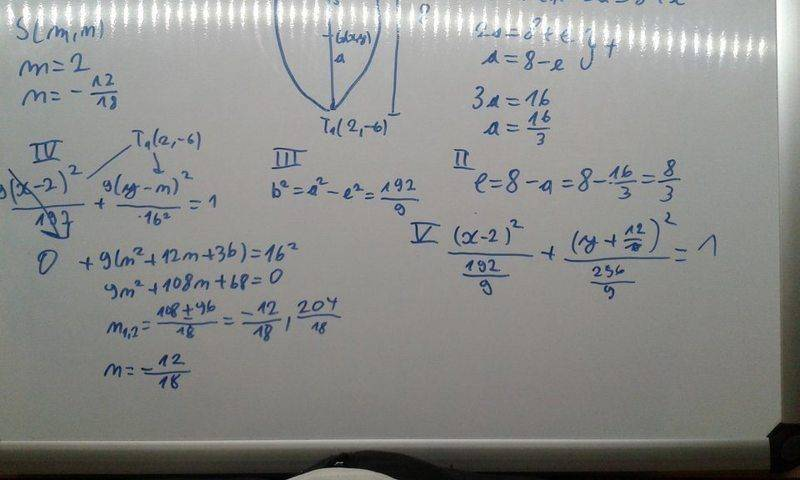So what I tried doing was:
From the definition I know that the sum of the distance from each of the two fucoses to the outer line is constant and it equals 2a. We are also told that a+e=8. From the picture we can clearly see that the distance from the upper tocus to the vertex equals a+e( or 8). And we also know that 2a=8+e solving both equation we get that a=16/3 and e=8/3. Then I used the pitagoras therom to get b( the minor axis) and from then on I used the point (2,-6) in the ellipse equation to get the center( later I realized that I could just substract e from the first focus). I got the final equation, however when I go to check it with the point (26/5,2) I don't get the right answer.
Below I will include the picture of the ellipse I made using the desmos graphing calculator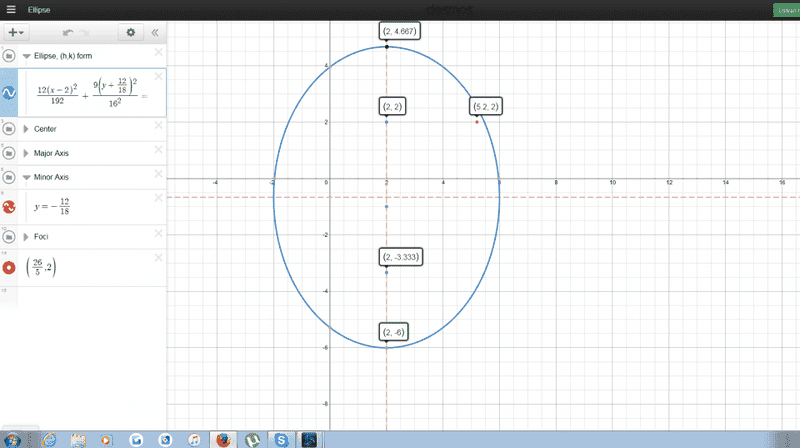.
as you can see the point does not lay on the line.
I would really appreciate it if you could check my work and find my mistake.

I didn't check your calculations, but do you know for certain that the ellipse is in a horizontal or vertical orientation, i.e. that there is no xy term in your general form that first needs to be removed with a rotation of axes?

I didn't check your calculations, but do you know for certain that the ellipse is in a horizontal or vertical orientation, i.e. that there is no xy term in your general form that first needs to be removed with a rotation of axes?
In fact, I think the answer to my question is that it is not rotated, because the focus is at (2,2) and a vertex at (2,-6). I'll need to check the algebra further... It appears it is in a vertical orientation. ...editing... some calculations I did give a=b=8 as one solution, making it a circle, with c=0. I will need to check my algebra. I would be a little surprised if this is the case. In any case, this problem appears to be somewhat challenging...editing some more... the info given of the focus position, vertex position, along with a+e=8 results in a cubic equation for "b" and one solution was b=8, for focus (2,2) being the top or bottom focus, and in both cases, the other solutions were complex/imaginary. The resulting circle does not pass through the point (26/5,2). Thereby the result I obtained is that the info given in the original problem is inconsistent. (I'm using eccentricity e=c/b and c^2=b^2-a^2. Even though it's been a while since I studied the ellipse in detail, I think I got this part correct.)

Last edited:
•Matejxx1
I haven't managed to figure out how you obtain 2a=8+e.

•Matejxx1

•Matejxx1
I would also like to add, that I noticed you guys use c for the distance between the focus and the center of the ellipse. I was unclear my mistake. We denote e as the distance between the focus and the center. So basically our e is your c

I didn't check your calculations, but do you know for certain that the ellipse is in a horizontal or vertical orientation, i.e. that there is no xy term in your general form that first needs to be removed with a rotation of axes?
This is a high school problem and they have not done any kind of problem where the ellipse's axis are parallel to x and y and as you said the focus and the vertex are both on x=2

The resulting circle does not pass through the point (26/5,2). Thereby the result I obtained is that the info given in the original problem is inconsistent. (I'm using eccentricity e=c/b and c^2=b^2-a^2. Even though it's been a while since I studied the ellipse in detail, I think I got this part correct.)
I got many different answers as well. Once I got that it's just a lie with a=8 and b =0

haruspex said:
I haven't managed to figure out how you obtain 2a=8+e.
Like I mentioned at the top. I was a little unclear. What I mean by e is the distance between the focus and the center ( basically your c)
and the distance from |GT1|=8 and |G2T1|=a-e so the distance from both focuses to the point T1 is 8+a-e and this equals 2a. Aha . . . I see it know will try to do the problem again

Ok so now i spend quite a bit if time on this problem. I did it from the start again and this is what I got: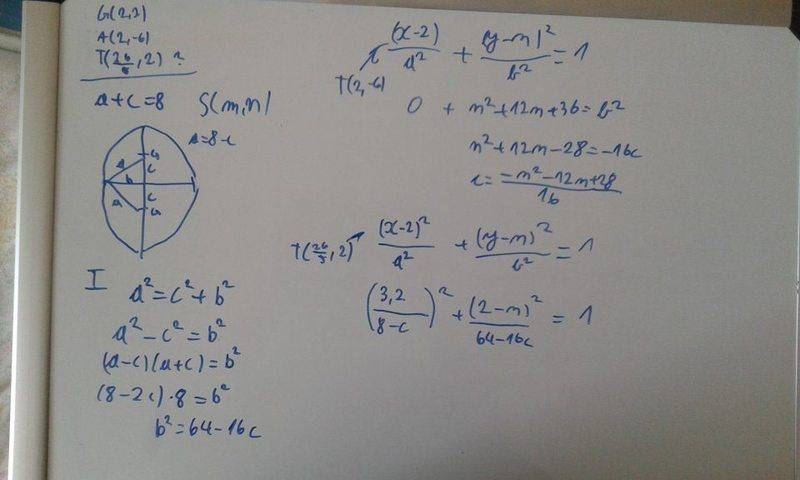then I used wolfram alpha to solve this equation and got this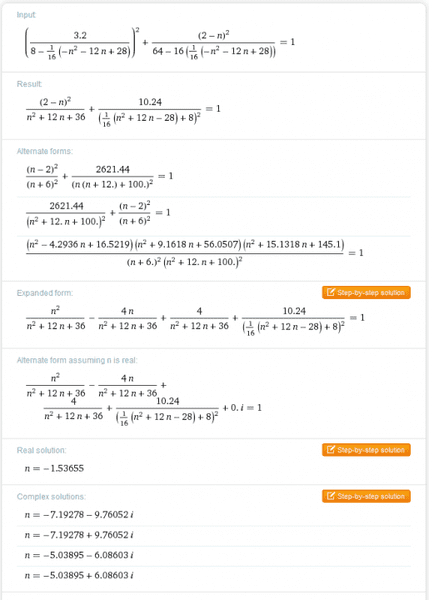only 1 soultion was real so I used that one to calculate both of the axis. However once again I got an ellipse but the point (26/5,2) was not on it. Do you guys think it's possible that the instruction are wrong because I'm completely lost at this point

Last edited:
Matejxx1 said:
Ok so now i spend quite a bit if time on this problem. I did it from the start again and this is what I got:
View attachment 100447
then I used wolfram alpha to solve this equation and got this
View attachment 100448
only 1 soultion was real so I used that one to calculate both of the axis. However once again I got an ellipse but the point (26/5,2) was on it. Do you guys think it's possible that the instruction are wrong because I'm completely lost at this point
I assume you meant to write that the point was not on it.
Without using the location of the point on it, you do not have enough information to find the equation, so I do not understand what you did.

•Matejxx1
Since the vertex is also a point on the ellipse, you know two points. You also know one focus, and the other focus must be collinear with the vertex and the first focus. It is not hard to find the position of the other focus from that... just Pythagoras.

•Matejxx1
No need to answer that. I found it . It was soo stupid . . .